Publicité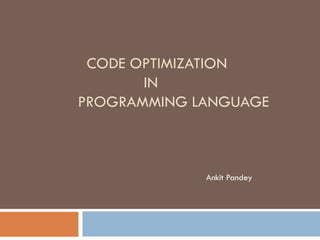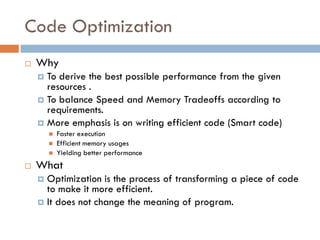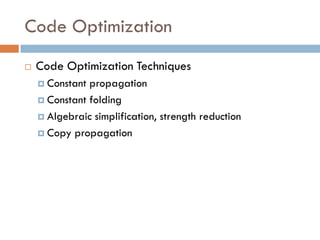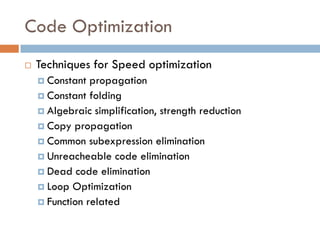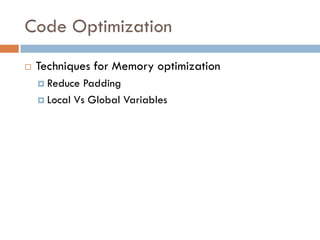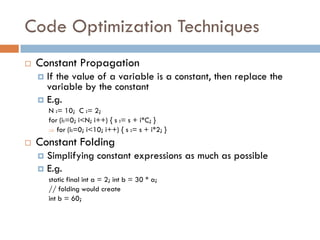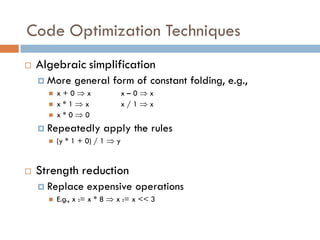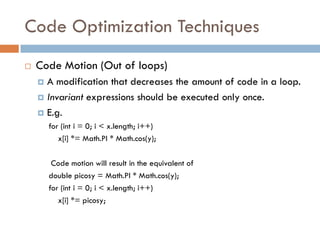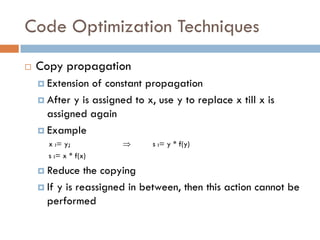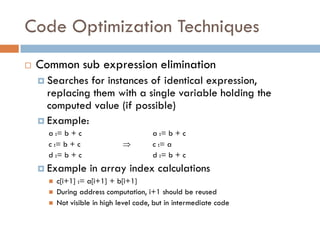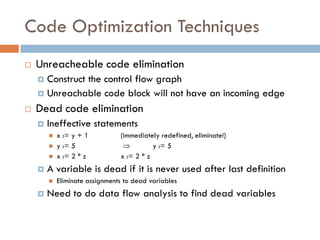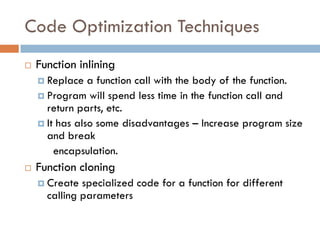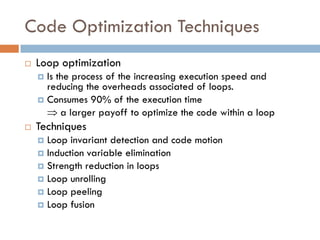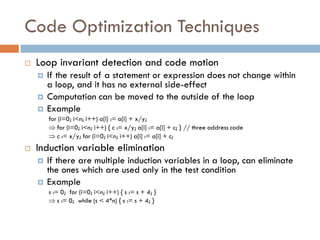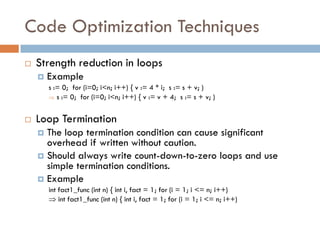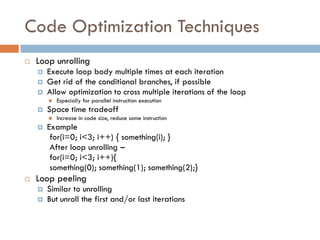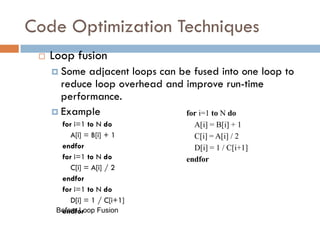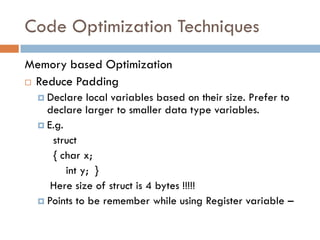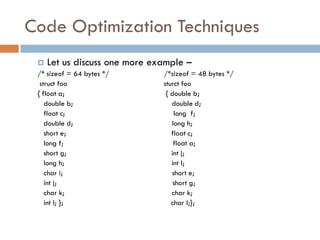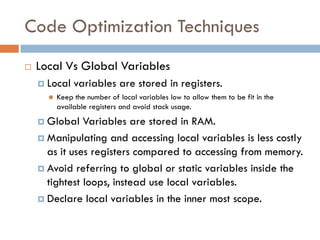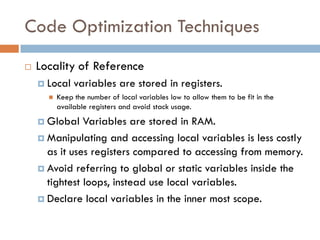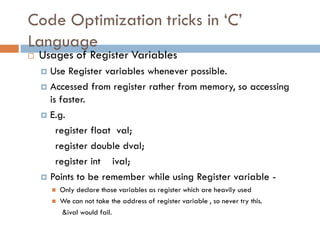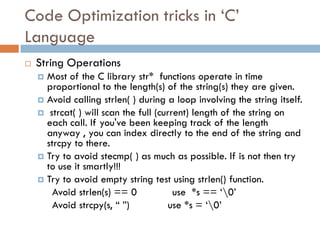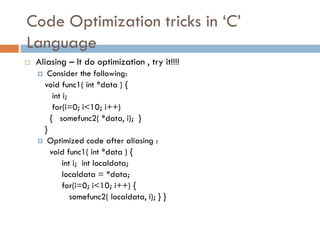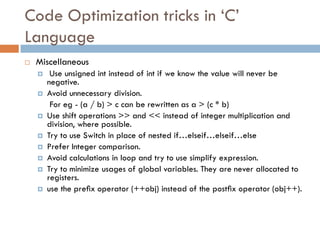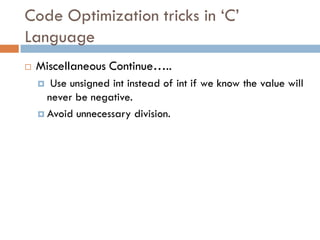1 sur 26
Publicité

### Optimization in Programming languages

1. CODE OPTIMIZATION IN PROGRAMMING LANGUAGE Ankit Pandey
2. Code Optimization  Why  To derive the best possible performance from the given resources .  To balance Speed and Memory Tradeoffs according to requirements.  More emphasis is on writing efficient code (Smart code)  Faster execution  Efficient memory usages  Yielding better performance  What  Optimization is the process of transforming a piece of code to make it more efficient.  It does not change the meaning of program.
3. Code Optimization  Code Optimization Techniques  Constant propagation  Constant folding  Algebraic simplification, strength reduction  Copy propagation
4. Code Optimization  Techniques for Speed optimization  Constant propagation  Constant folding  Algebraic simplification, strength reduction  Copy propagation  Common subexpression elimination  Unreacheable code elimination  Dead code elimination  Loop Optimization  Function related
5. Code Optimization  Techniques for Memory optimization  Reduce Padding  Local Vs Global Variables
6. Code Optimization Techniques  Constant Propagation  If the value of a variable is a constant, then replace the variable by the constant  E.g. N := 10; C := 2; for (i:=0; i<N; i++) { s := s + i*C; }  for (i:=0; i<10; i++) { s := s + i*2; }  Constant Folding  Simplifying constant expressions as much as possible  E.g. static final int a = 2; int b = 30 * a; // folding would create int b = 60;
7. Code Optimization Techniques  Algebraic simplification  More general form of constant folding, e.g.,  x + 0  x x – 0  x  x * 1  x x / 1  x  x * 0  0  Repeatedly apply the rules  (y * 1 + 0) / 1  y  Strength reduction  Replace expensive operations  E.g., x := x * 8  x := x << 3
8. Code Optimization Techniques  Code Motion (Out of loops)  A modification that decreases the amount of code in a loop.  Invariant expressions should be executed only once.  E.g. for (int i = 0; i < x.length; i++) x[i] *= Math.PI * Math.cos(y); Code motion will result in the equivalent of double picosy = Math.PI * Math.cos(y); for (int i = 0; i < x.length; i++) x[i] *= picosy;
9. Code Optimization Techniques  Copy propagation  Extension of constant propagation  After y is assigned to x, use y to replace x till x is assigned again  Example x := y;  s := y * f(y) s := x * f(x)  Reduce the copying  If y is reassigned in between, then this action cannot be performed
10. Code Optimization Techniques  Common sub expression elimination  Searches for instances of identical expression, replacing them with a single variable holding the computed value (if possible)  Example: a := b + c a := b + c c := b + c  c := a d := b + c d := b + c  Example in array index calculations  c[i+1] := a[i+1] + b[i+1]  During address computation, i+1 should be reused  Not visible in high level code, but in intermediate code
11. Code Optimization Techniques  Unreacheable code elimination  Construct the control flow graph  Unreachable code block will not have an incoming edge  Dead code elimination  Ineffective statements  x := y + 1 (immediately redefined, eliminate!)  y := 5  y := 5  x := 2 * z x := 2 * z  A variable is dead if it is never used after last definition  Eliminate assignments to dead variables  Need to do data flow analysis to find dead variables
12. Code Optimization Techniques  Function inlining  Replace a function call with the body of the function.  Program will spend less time in the function call and return parts, etc.  It has also some disadvantages – Increase program size and break encapsulation.  Function cloning  Create specialized code for a function for different calling parameters
13. Code Optimization Techniques  Loop optimization  Is the process of the increasing execution speed and reducing the overheads associated of loops.  Consumes 90% of the execution time  a larger payoff to optimize the code within a loop  Techniques  Loop invariant detection and code motion  Induction variable elimination  Strength reduction in loops  Loop unrolling  Loop peeling  Loop fusion
14. Code Optimization Techniques  Loop invariant detection and code motion  If the result of a statement or expression does not change within a loop, and it has no external side-effect  Computation can be moved to the outside of the loop  Example for (i=0; i<n; i++) a[i] := a[i] + x/y;  for (i=0; i<n; i++) { c := x/y; a[i] := a[i] + c; } // three address code  c := x/y; for (i=0; i<n; i++) a[i] := a[i] + c;  Induction variable elimination  If there are multiple induction variables in a loop, can eliminate the ones which are used only in the test condition  Example s := 0; for (i=0; i<n; i++) { s := s + 4; }  s := 0; while (s < 4*n) { s := s + 4; }
15. Code Optimization Techniques  Strength reduction in loops  Example s := 0; for (i=0; i<n; i++) { v := 4 * i; s := s + v; )  s := 0; for (i=0; i<n; i++) { v := v + 4; s := s + v; )  Loop Termination  The loop termination condition can cause significant overhead if written without caution.  Should always write count-down-to-zero loops and use simple termination conditions.  Example int fact1_func (int n) { int i, fact = 1; for (i = 1; i <= n; i++)  int fact1_func (int n) { int i, fact = 1; for (i = 1; i <= n; i++)
16. Code Optimization Techniques  Loop unrolling  Execute loop body multiple times at each iteration  Get rid of the conditional branches, if possible  Allow optimization to cross multiple iterations of the loop  Especially for parallel instruction execution  Space time tradeoff  Increase in code size, reduce some instruction  Example for(i=0; i<3; i++) { something(i); } After loop unrolling – for(i=0; i<3; i++){ something(0); something(1); something(2);}  Loop peeling  Similar to unrolling  But unroll the first and/or last iterations
17. Code Optimization Techniques  Loop fusion  Some adjacent loops can be fused into one loop to reduce loop overhead and improve run-time performance.  Example for i=1 to N do A[i] = B[i] + 1 endfor for i=1 to N do C[i] = A[i] / 2 endfor for i=1 to N do D[i] = 1 / C[i+1] endforBefore Loop Fusion for i=1 to N do A[i] = B[i] + 1 C[i] = A[i] / 2 D[i] = 1 / C[i+1] endfor
18. Code Optimization Techniques Memory based Optimization  Reduce Padding  Declare local variables based on their size. Prefer to declare larger to smaller data type variables.  E.g. struct { char x; int y; } Here size of struct is 4 bytes !!!!!  Points to be remember while using Register variable –
19. Code Optimization Techniques  Let us discuss one more example – /* sizeof = 64 bytes */ /*sizeof = 48 bytes */ struct foo sturct foo { float a; { double b; double b; double d; float c; long f; double d; long h; short e; float c; long f; float a; short g; int j; long h; int l; char i; short e; int j; short g; char k; char k; int l; }; char I;};
20. Code Optimization Techniques  Local Vs Global Variables  Local variables are stored in registers.  Keep the number of local variables low to allow them to be fit in the available registers and avoid stack usage.  Global Variables are stored in RAM.  Manipulating and accessing local variables is less costly as it uses registers compared to accessing from memory.  Avoid referring to global or static variables inside the tightest loops, instead use local variables.  Declare local variables in the inner most scope.
21. Code Optimization Techniques  Locality of Reference  Local variables are stored in registers.  Keep the number of local variables low to allow them to be fit in the available registers and avoid stack usage.  Global Variables are stored in RAM.  Manipulating and accessing local variables is less costly as it uses registers compared to accessing from memory.  Avoid referring to global or static variables inside the tightest loops, instead use local variables.  Declare local variables in the inner most scope.
22. Code Optimization tricks in „C‟ Language  Usages of Register Variables  Use Register variables whenever possible.  Accessed from register rather from memory, so accessing is faster.  E.g. register float val; register double dval; register int ival;  Points to be remember while using Register variable -  Only declare those variables as register which are heavily used  We can not take the address of register variable , so never try this. &ival would fail.
23. Code Optimization tricks in „C‟ Language  String Operations  Most of the C library str* functions operate in time proportional to the length(s) of the string(s) they are given.  Avoid calling strlen( ) during a loop involving the string itself.  strcat( ) will scan the full (current) length of the string on each call. If you've been keeping track of the length anyway , you can index directly to the end of the string and strcpy to there.  Try to avoid stecmp( ) as much as possible. If is not then try to use it smartly!!!  Try to avoid empty string test using strlen() function. Avoid strlen(s) == 0 use *s == „0‟ Avoid strcpy(s, “ ”) use *s = „0‟
24. Code Optimization tricks in „C‟ Language  Aliasing – It do optimization , try it!!!!  Consider the following: void func1( int *data ) { int i; for(i=0; i<10; i++) { somefunc2( *data, i); } }  Optimized code after aliasing : void func1( int *data ) { int i; int localdata; localdata = *data; for(i=0; i<10; i++) { somefunc2( localdata, i); } }
25. Code Optimization tricks in „C‟ Language  Miscellaneous  Use unsigned int instead of int if we know the value will never be negative.  Avoid unnecessary division. For eg - (a / b) > c can be rewritten as a > (c * b)  Use shift operations >> and << instead of integer multiplication and division, where possible.  Try to use Switch in place of nested if…elseif…elseif…else  Prefer Integer comparison.  Avoid calculations in loop and try to use simplify expression.  Try to minimize usages of global variables. They are never allocated to registers.  use the preﬁx operator (++obj) instead of the postﬁx operator (obj++).
26. Code Optimization tricks in „C‟ Language  Miscellaneous Continue…..  Use unsigned int instead of int if we know the value will never be negative.  Avoid unnecessary division.
Publicité